# Excel DAX - Deleting a Calculated Field

You can delete both explicit and implicit calculated fields. There are several ways of doing so, which you will learn in this chapter.

However, you need to remember the following points before deleting a calculated field −

• An explicit calculated field can be used in more than one PivotTable and/or PivotChart. Hence, you need to make sure that deleting the explicit calculated field does not affect any of the reports that you have already generated.

• An explicit calculated field can be used in the calculations of other explicit calculated fields. Hence, you need to make sure that the explicit calculated field is not used in any of the calculations of other explicit calculated fields.

• An implicit calculated field is limited to the PivotTable or PivotChart where it is used. Hence, before deleting an implicit calculated field, it is just sufficient to make sure that it can be deleted from the corresponding PivotTable or PivotChart.

• Creating an implicit calculated field is simpler than creating an explicit calculated field. Hence, more caution is required before deleting an explicit calculated field.

• You cannot create an explicit calculated field, if the name conflicts with the name of an implicit calculated field. Hence, you might have to delete that implicit calculated field before creating the explicit calculated field.

## Deleting an Explicit Calculated Field in the Data Model

You can delete an explicit calculated field either in the data view or the diagram view in the Data Model.

### Deleting an Explicit Calculated Field in the Data View

• Locate the calculated field in the calculations area in the Data View.
• Right-click the calculated field.
• Click Delete in the dropdown list.Message appears for delete confirmation.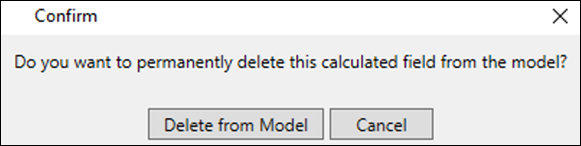Click Delete from Model. The explicit calculated field will get deleted.

### Deleting an Explicit Calculated Field in the Diagram View

• Locate the calculated field in the data table in the Diagram View.
• Right-click the calculated field name.
• Click Delete in the dropdown list.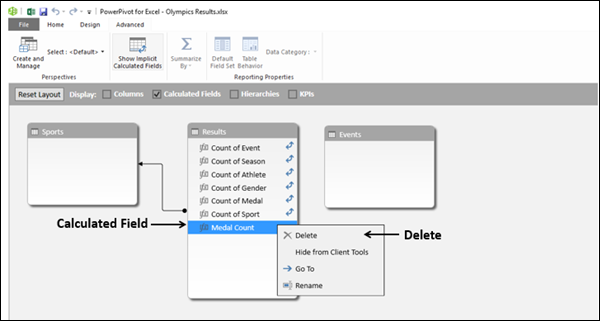Message appears for delete confirmation.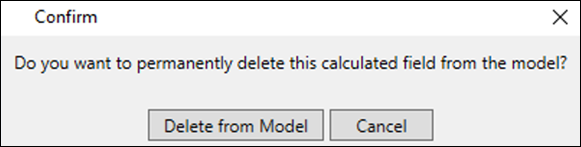Click Delete from Model. The explicit calculated field will get deleted and it will not be seen in the fields list of the data table.

### Deleting an Explicit Calculated Field in the Excel Window

You can delete an explicit calculated field from the Excel window as follows −

• Click the POWERPIVOT tab on the Ribbon.
• Click Calculated Field in the Calculations group.
• Click Manage Calculated Fields in the dropdown list.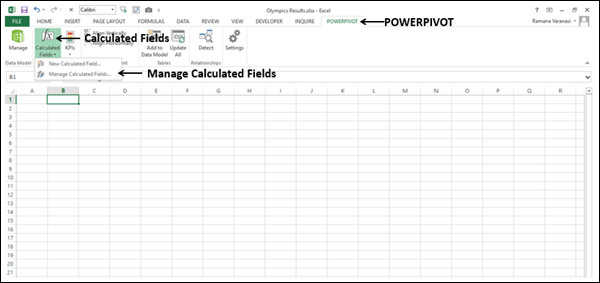Manage Calculated Fields dialog box appears.

• Click the explicit calculated field name.
• Click the Delete button.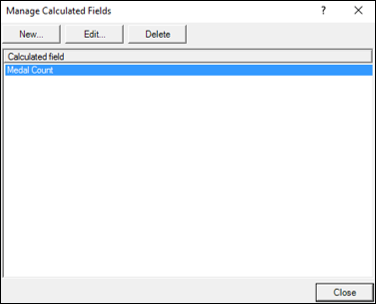Confirmation message for deletion appears.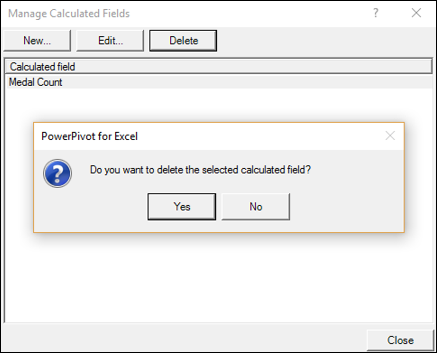• Click Yes. Information message that the Data Model is changed appears at the top.
• Click the Close button in the dialog box.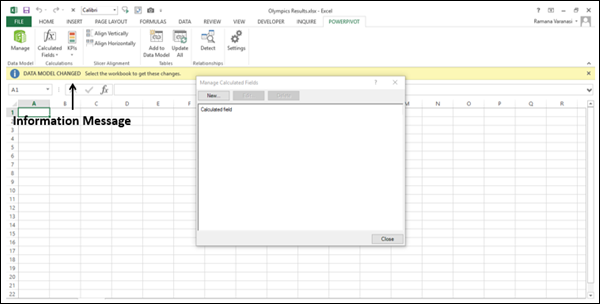The explicit calculated field will get deleted and it will not be seen in the PivotTable/PivotChart Fields list in the workbook.

## Deleting an Implicit Calculated Field

You can delete an implicit calculated field either in the data view or the diagram view in the Data Model.

### Deleting an Implicit Calculated Field in the Data View

• Locate the calculated field in the calculations area in the Data View.
• Right-click the calculated field.
• Click Delete in the dropdown list.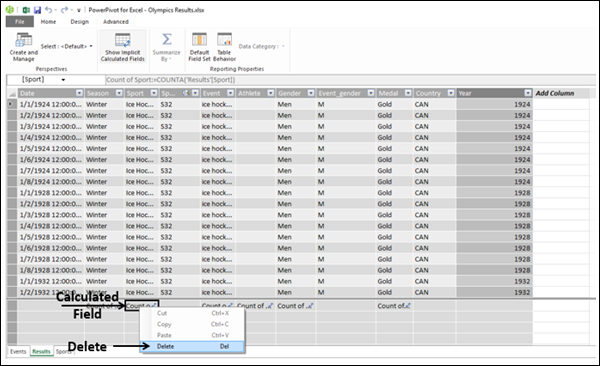Message appears for delete confirmation.• Click Delete from Model. The implicit calculated field will get deleted.

### Deleting an Implicit Calculated Field in the Diagram View

• Locate the calculated field in the data table in the Diagram View.
• Right-click the calculated field name.
• Click Delete in the dropdown list.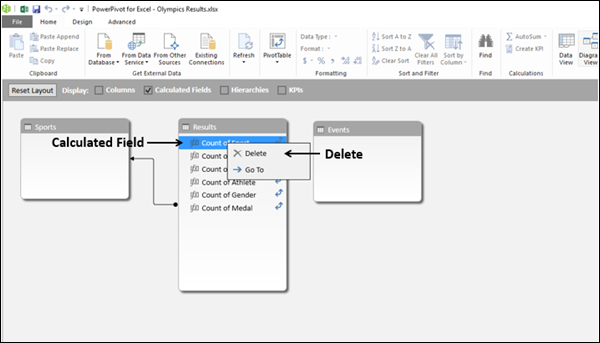Message appears for delete confirmation.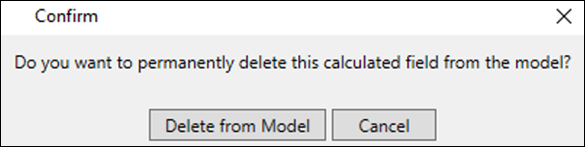Click Delete from Model. The implicit calculated field will get deleted and it will not be seen in the fields list of the data table.